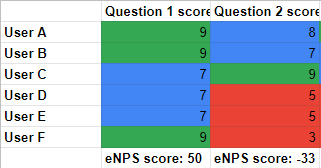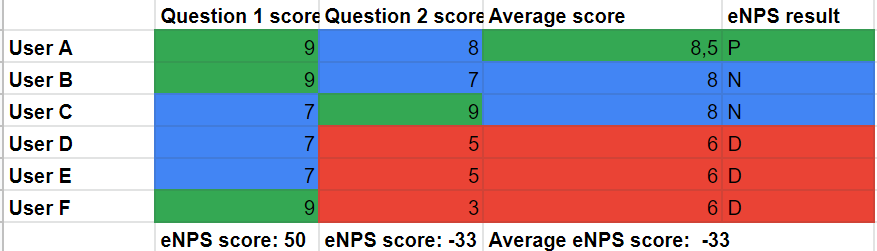# How is the eNPS score calculated?

eNPS stands for Employee Net Promoter Score. The eNPS score is a management tool that can be used to measure employee loyalty. This score can be determined using one or more questions.

For eNPS, a ten-point scale (0-1-2-3-4-5-6-7-8-9-10) is used:

• Promoters (fans) give a 9 or 10: they have a positive influence on the eNPS score.
• Neutral employees give a 7 or 8: they have no influence on the eNPS score.
• Critics (or dissatisfied employees) give a 6 or lower: they have a negative influence on the eNPS score.

The eNPS score is expressed on a scale of -100 to +100, where anything above 0 can be considered acceptable. If you score between 10 and 30, you are doing well. If you score above 50, you are even doing excellent.

Note: This is not a percentage - eNPS scores are calculated using a weighting matrix. Below, we have provided an example that explains how this score is calculated.

### Calculating the eNPS score from a single question

To calculate the eNPS score, we assign values to promoters, neutral employees, and critics.

• A promoter (9 or 10) receives a value of +1.
• A neutral employee (7 or 8) receives a value of 0.
• A critic (6 or lower) receives a value of -1.

The final score is calculated by adding up all the values and dividing them by the total number of employees who answered the question, multiplied by 100.

In the table below, you can see an example of eNPS results. Question 1 has an eNPS score of 50, and Question 2 has an eNPS score of -33. We will explain how we came at these scores for each question.Question 1:

The eNPS score for the first question is 50. A total of six employees answered this question. Three of them gave a score of 9 (promoters), and three gave a score of 7 (neutral employees). In terms of values, you have three times +1 (promoters) and three times 0 (neutral employees). This makes the total value +3.

Therefore, the eNPS score is 3/6 x 100 = 50.

Question 2:

The eNPS score for the second question is -33. A total of six employees answered this question. You have one promoter, two neutral employees, and three critics. In terms of values, you have one time +1 (promoters), two times 0 (neutral employees), and three times -1 (critics). This makes the total value -2.

Therefore, the eNPS score is -2/6 x 100 = -33.

### Calculating the average eNPS score

You do not calculate the average eNPS score by taking the average of the individual eNPS scores. In the example, you can see that the average eNPS score is -33, even though the score for Question 1 is 50 and the score for Question 2 is -33. The average eNPS score is calculated by taking the average of the actual scores per question. Then, it is determined whether the average score is a promoter, a neutral employee, or a critic.

Example:

To explain how we calculate the average eNPS score, we have added a new column to the table, '

Average score.' This column shows the average score of questions 1 and 2 combined.

• User A has a score of 8.5 because it is the average of 9 + 8.
• User F has a score of 6 because it is the average of 9 + 3.

Based on these average scores, it is determined again whether an employee is a promoter, a neutral employee, or a critic. Then, these values are used again to calculate the eNPS score. In this example, there is one promoter (+1), two neutral employees (0), and three critics (3x +1).

Therefore, the eNPS score is -2/6 x 100 = -33.